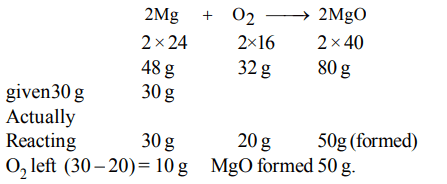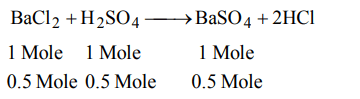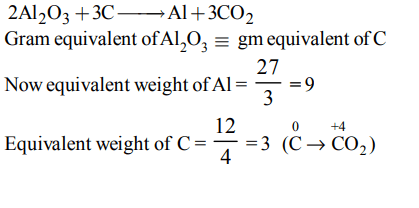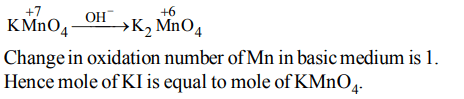## Some Basic Concepts of Chemistry Questions and Answers Part-5

1. 30 g of Magnesium and 30 g of oxygen are reacted, then the residual mixture contains
a) 50 g of Magnesium oxide and 10 g of oxygen
b) 40 g of Magnesium oxide and 20 g of oxygen
c) 45 g of Magnesium oxide and 15 g of oxygen
d) 60 g of Magnesium oxide only

Explanation:2. In order to prepare one litre normal solution of $KMnO_{4}$, how many grams of $KMnO_{4}$  are required if the solution is to be used in acid medium for oxidation?
a) 158 g
b) 62.0 g
c) 31.6 g
d) 790 g

Explanation: Eq. wt of KMnO4 in acid medium is 31.6 g. Hence this much amount must be dissolved in 1 litre to prepare normal solution

3. In a chemical reaction
$K_{2}Cr_{2}O_{7}+xH_{2}SO_{4}+ySO_{2}\rightarrow K_{2}SO_{4}+zCr_{2}\left(SO_{4}\right)_{3}+H_{2}O$
the values of x, y, z are
a) 4, 1, 4
b) 1, 3, 1
c) 3, 2, 3
d) 2, 1, 2

Explanation:4. The maximum amount of  $BaSO_{4}$  precipitated on mixing equal volumes of $BaCI_{2}\left(0.5M\right)$   with $H_{2}SO_{4}\left(1M\right)$   will correspond to
a) 1.0 M
b) 0.5 M
c) 1.5 M
d) 2.0 M

Explanation:5. In the reaction  $4NH_{3}\left(g\right)+5O_{2}\left(g\right)\rightarrow4NO\left(g\right)+6H_{2}O\left(l\right)$         , when 1 mole of ammonia and 1 mole of O2 are made to react to completion
a) 1.0 mole of $H_{2}O$  is produced
b) 1.0 mole of NO will be produced
c) all the ammonia will be consumed
d) all the oxygen will be consumed

Explanation:All, O2 consumed being limiting.

6. The set of numerical coefficients that balances the equation$K_{2}CrO_{4}+HCI\rightarrow K_{2}Cr_{2}O_{7}+KCI+H_{2}O$         is
a) 2, 2, 1, 2, 1
b) 2, 2, 1, 1, 1
c) 2, 1, 1, 2, 1
d) 1, 1, 2, 2, 1

Explanation:7. The mass of carbon anode consumed (giving only carbondioxide) in the production of 270 kg of aluminium metal from bauxite by the Hall process is (Atomic mass: Al = 27)
a) 270 kg
b) 540 kg
c) 90 kg
d) 180 kg

Explanation:8. The number of moles of  $KMnO_{4}$  reduced by one mole of KI in alkaline medium is:
a) one
b) two
c) five
d) one fifth

Explanation:9. Volume occupied by one molecule of water $\left(density = 1g cm^{-3}\right)$     is :
a) $9.0 × 10^{-23}cm^{3}$
b) $6.023 × 10^{-23}cm^{3}$
c) $3.0 × 10^{-23}cm^{3}$
d) $5.5 × 10^{-23}cm^{3}$10. Number of moles of  $MnO_4^-$  required to oxidize one mole of ferrous oxalate completely in acidic medium will be